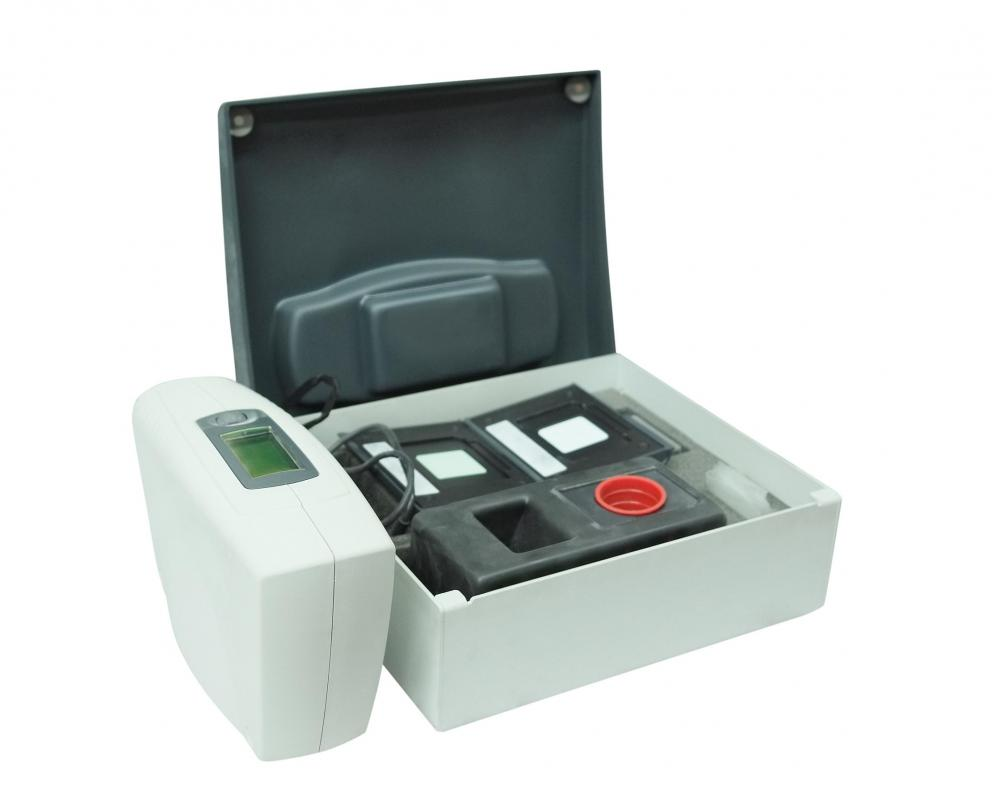# What Is a Calibration Curve?

E.A. Sanker
E.A. SankerSpectrophotometry can be useful in determining the concentration of an unknown solution.

A calibration curve is a method used in analytical chemistry to determine the concentration of an unknown sample solution. It is a graph generated by experimental means, with the concentration of solution plotted on the x-axis and the observable variable — for example, the solution’s absorbance — plotted on the y-axis. The curve is constructed by measuring the concentration and absorbance of several prepared solutions, called calibration standards. Once the curve has been plotted, the concentration of the unknown solution can be determined by placing it on the curve based on its absorbance or other observable variable.

Chemical solutions absorb different amounts of light based on their concentration. This fact is quantified in an equation known as Beer’s law, which shows a linear relation between a solution’s light absorbance and its concentration. Researchers can measure the absorbance of a solution using a laboratory instrument called a spectrophotometer. This process as a whole is called spectrophotometry.

Spectrophotometry can be useful in determining the concentration of an unknown solution. For example, if a researcher has a sample of river water and wants to know its lead content, he or she can determine it by using a spectrophotometer to plot a calibration curve. First, the researcher creates several standard solutions of lead, ranging from less to more concentrated. These samples are placed into the spectrophotometer, which records a different absorbance for each one.

The experimentally determined absorbance values are plotted on a graph against the known concentration of each calibration standard. A set of points is created, which in the case of absorbance should be roughly linear due to Beer’s law. A line is drawn to connect these data points, forming the calibration curve. In almost every case, the data points will not be mathematically exact, so the line should be drawn to intercept the maximum number of points — it is a line of best fit. Although the relationship of absorbance to concentration is linear, this is not always true for other experimentally determined variables, and occasionally curves must be employed to describe the relationship.

At this stage, the unknown solution can be analyzed. The sample is inserted into the spectrophotometer, and its absorbance is measured. Since this sample is being measured against several standards containing the same compound, its absorbance and concentration must fall somewhere along the calibration curve for that compound. This means that once the solution's absorbance is known, its concentration can be deduced mathematically or graphically.

A horizontal line can be drawn from the unknown solution’s y-value — its absorbance, which has just been measured. The point at which the line crosses the calibration curve will indicate the x-value — the concentration. A vertical line, drawn downwards from this point, gives the concentration of the unknown solution. The equation for the line of the calibration curve can also be used to mathematically determine the solution's concentration.

## You might also Likeanon994504

What is the differences between standard curve and calibration curve?

anon963578

Thank you! I found the text very clear and informative. This will definitely help me get through my chemistry practicals with ease. Very much appreciated.

anon937657

What is the y-intercept for?

Realited
Can this even be considered viable? If there a constant, and a variable, how can data be gathered, and be considered reliable and accurate? Just wondering is all.
eoconnor
If there is an X and a Y axis involved in this equation, we can surmise that this is similar to calculus, no? Or was it trigonometry? I'm almost certain I've heard of the X/Y axis someplace else.
Grinderry

Thank You! I'm glad that this article was written, as I have been looking fora more complete and thorough explanation of a calibration curve for a long time. Most of the other sites I've gone to have given more technical jargon than actual info. I will be able to use this data for my school projects.